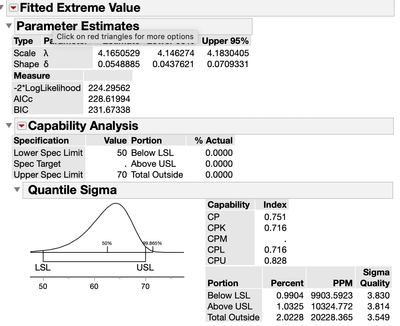Choose Language Hide Translation Bar
Highlighted

## Extreme Value Continuous Fit Documentation?

I'm looking for some information on the "Extreme Value" distribution type, found in the Distribution Platform under Continuous Fit.  Specifically, what is the formula for this cumulative distribution?  Here's an example:

``````Names Default To Here(1);
dt = Open("\$SAMPLE_DATA/Big Class.jmp");
Distribution(
Continuous Distribution(
Column(:height),
Quantiles(0),
Histogram(0),
Vertical(0),
Outlier Box Plot(0),
Normal Quantile Plot(1),
Fit Distribution(Extreme Value(Spec Limits(LSL(50), USL(70))))
)
);``````

Here's a pic of the fit in the Distribution report.  There are two parameters, lambda and delta.I checked the "JSL Syntax Reference > > Probability Functions".  The closest functions I can see are the "SEV Distribution" and "LEV Distribution", which both also take 2 parameters in addition to the independent variable x.

However, the values I get using either of these functions with the parameters in the Distribution report do not jibe.  E.G: at my LSL of 50, the report says 0.9904% of the distrubution is below my lower limit.  However:

``````x = 50;					//my LSL
mu = 4.1650529;			//taken from report
sigma = 0.0548885;		//taken from report
pLEV = LEV Distribution(x, mu, sigma);
pSEV = SEV Distribution(x, mu, sigma);
Show(pLEV, pSEV);``````

Both functions return a probability of 100%.  In fact, they remain 100% unless I lower x to ~4.   So clearly either these aren't the right functions or I'm missing something.

Any ideas?

1 ACCEPTED SOLUTION

Accepted Solutions
Highlighted

## Re: Extreme Value Continuous Fit Documentation?

See this part of the Help system: Continuous Fit Distributions.

You find the information yourself. Select Tools > Help and click on the Fitted Extreme Value outline in Distribution platform. It will take you to the Help for this model. The first page is an overview but it contains a link to the statistical details that you want.

Learn it once, use it forever!
3 REPLIES 3
Highlighted

## Re: Extreme Value Continuous Fit Documentation?

See this part of the Help system: Continuous Fit Distributions.

You find the information yourself. Select Tools > Help and click on the Fitted Extreme Value outline in Distribution platform. It will take you to the Help for this model. The first page is an overview but it contains a link to the statistical details that you want.

Learn it once, use it forever!
Highlighted

## Re: Extreme Value Continuous Fit Documentation?

Thanks Mark.  That's the answer.  For benefit of others, the necessary transforms are shown below:

``````x = 70;					//my LSL
lambda = 4.1650529;		//taken from report
delta = 0.0548885;		//taken from report
alpha = Exp(lambda);	//scale
beta = 1/delta;			//shape
pex = Weibull Distribution(x, beta, alpha);
pex2 = 1-Exp(-(x/alpha)^beta);		//taken from wiki as a confirmation
Show(pex, pex2);``````

Sidenote: Interesting that when I clicked on "Continuous Fit Distributions" in the JMP Help Search Results, it just goes to a blank page.  In case anyone has the same issue, try opening the page as a separate window.  Might be my OS (Mac) or my browser (Safari).

Highlighted